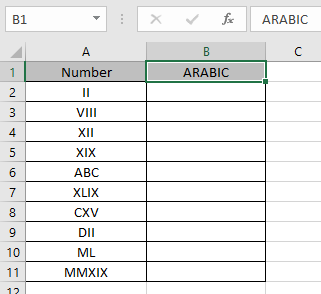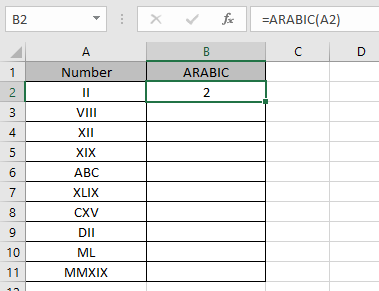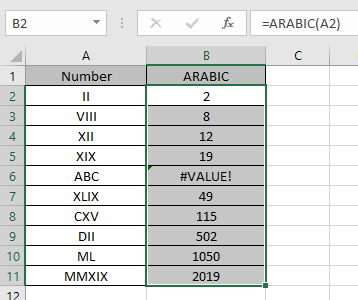# How to use the ARABIC Function in Excel

ARABIC function is a mathematical conversion operator used to convert the roman numerals as text to ARABIC numerals. ARABIC numerals to ROMAN numerals are shown below.

 ARABIC ROMAN 1 I 5 V 10 X 50 L 100 C 500 D 1000 M

The ARABIC function converts roman numeral as text to ARABIC numeral.
Syntax:

=ARABIC (text)

text : ROMAN numeral as text
Let’s understand this function using it in an example.Here we have some values which we will be providing to the function and to see what the function returns.
Use the formula:

=ARABIC (A2)

A2 : roman numeral as text (max upto 255 characters.)
Here argument to the formula is taken as cell reference.As you can see the formula converts from ROMAN numeral as text to the output arabic numeral representation.

Now copy the formula in other cells using Ctrl + D shortcut key.ABC is not a ROMAN numeral. So it returns the #VALUE! error.

These are the results returned by the ARABIC function.

As you can see all the input arabic numerals gets converted to ARABIC as text.

Use ARABIC function with other function to get results.

Notes:

1. The function returns #VALUE! Error, if the argument to the function is non ROMAN numeral text given in as cell reference.
2. The text cannot be more than 255 characters
3. The ARABIC function does the opposite of the ROMAN function in excel.

Hope you understood how to use ARABIC function and referring cell in Excel. Explore more articles on Excel function here. Please feel free to state your query or feedback for the above article.

Related Articles:

How to use the ROMAN Function in Excel

Popular Articles:

50 Excel Shortcuts to Increase Your Productivity

How to use the VLOOKUP Function in Excel

How to use the COUNTIF in Excel 2016

How to use the SUMIF Function in Excel

Terms and Conditions of use

The applications/code on this site are distributed as is and without warranties or liability. In no event shall the owner of the copyrights, or the authors of the applications/code be liable for any loss of profit, any problems or any damage resulting from the use or evaluation of the applications/code.